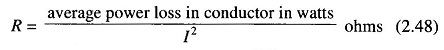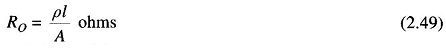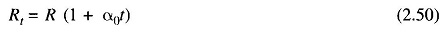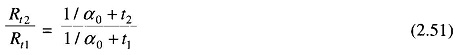## AC Resistance and DC Resistance Relation:

Though the contribution of line resistance to series line impedance can be neglected in most cases, it is the main source of line power loss. Thus while considering transmission line economy, the presence of line resistance must be considered. The effective AC resistance is given byWhere

• I is the rms current in the conductor in amperes.

Ohmic or DC resistance is given by the formulawhere

• ρ = resistivity of the conductor, ohm-m
• l = length, m
• A = cross-sectional area, m2

The effective resistances given by Eq. (2.48) is equal to the DC resistances of the conductor given by Eq. (2.49) only if the current distribution is uniform throughout the conductor.

For small changes in temperature, the resistances increases with temperature in accordance with the relationshipwhere

• R = resistances at temperature 0°C
• α0 = temperature coefficient of the conductor at 0°C

Equation (2.50) can be used to find the resistance Rt2 at a temperature t2, if resistance Rt1 at temperature t1 is knownScroll to Top